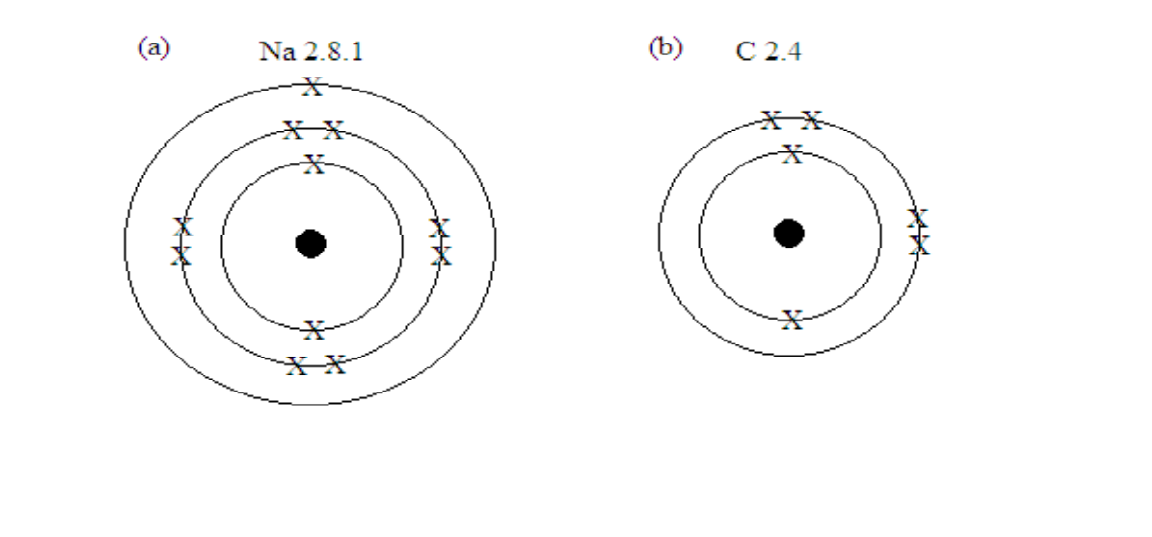# Atomic Structure and Periodic Table

An atom is the smallest particle of an element which takes part in a chemical reaction.

Atoms are electrically neutral. They have no overall charge. This is because number of protons is equal to number of electrons.

#### Fundamental particles of an atom

##### Electron
• It is a negatively charged particle.
• It has a charge of -1
• It is found in shells of around the nucleus of an atom
• It has a mass of 1/1840 atomic mass units (a.m.u)
##### Proton
• It is a positively charged particle
• It has a charge of +1
• It is found in the nucleus of an atom
• It has a mass of 1 atomic mass units (a.m.u)
##### Neutron
• It is a neutral particle
• It has no charge
• It is found in the nucleus of an atom
• It has a mass of 1 atomic mass units (a.m.u)

#### The diagram below represents an atom of lithium.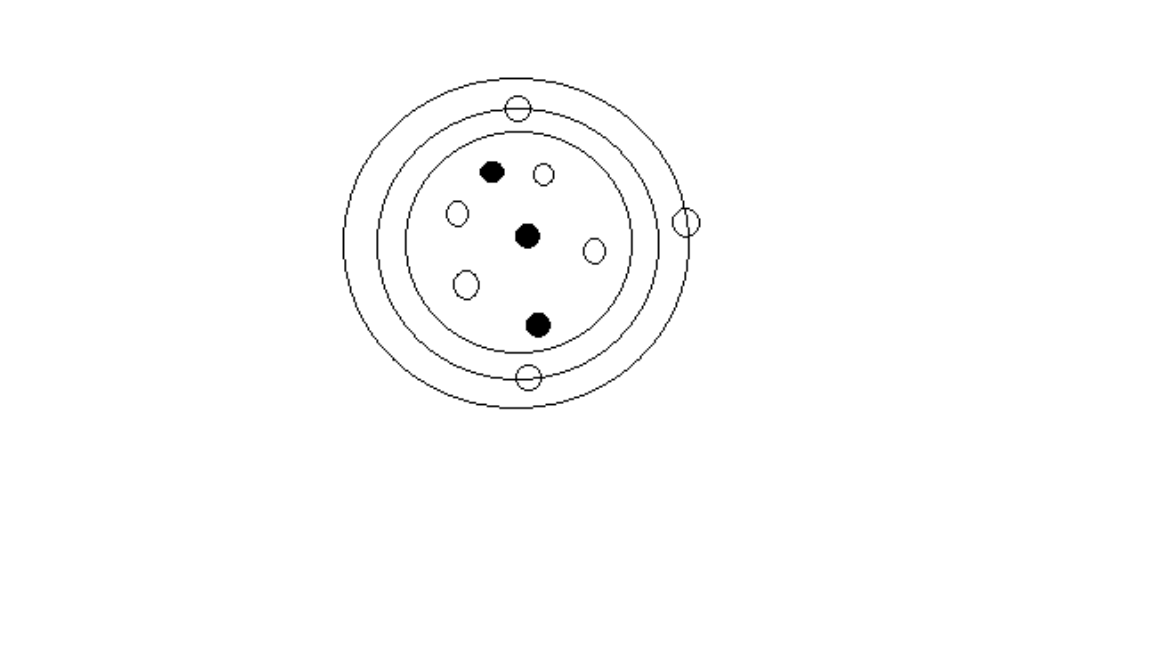##### The diagram interpretation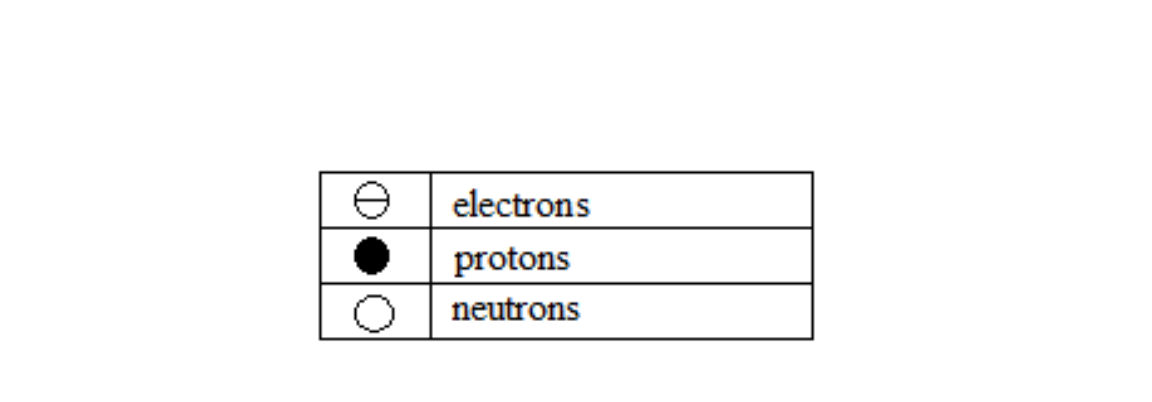###### Proton number

Proton number : It is the number of protons in the nucleus of an atom

Alternative term: Atomic number

Symbol: Z

###### Mass number

Mass number It the sum of protons and neutrons in the nucleus of an atom

Formula: A = Z + N

A = mass number

Z = proton (atomic number)

N = number of neutrons

#### Example 1

An atom of sodium has 11 protons and 12 neutrons. Calculate its mass number.

Solution

Formula: A = Z + N

Given that A = ? , Z = 11 and N = 12

A = 11 + 12

#### Example 2

An atom of aluminium has a mass number of 27 and has 13 protons. Work out the number of neutrons.

Solution

Formula: A = Z + N

Given that A = 27, Z = 13 and N = ?

27 = 13 + N

N = 27 - 13

#### Atomic numbers and mass numbers for the first 20 elements##### Nuclides

A nuclide consists of a symbol with the atomic number in front of the symbol and just below it and the mass number also in front and just above the symbol.

Nuclide notation: A = mass number, X = symbol of an atom, Z = proton (atomic number)

#### Isotopes

Isotopes are atoms of the same element having the same number of protons but different number of neutrons (and mass numbers)

##### Carbon has three isotopes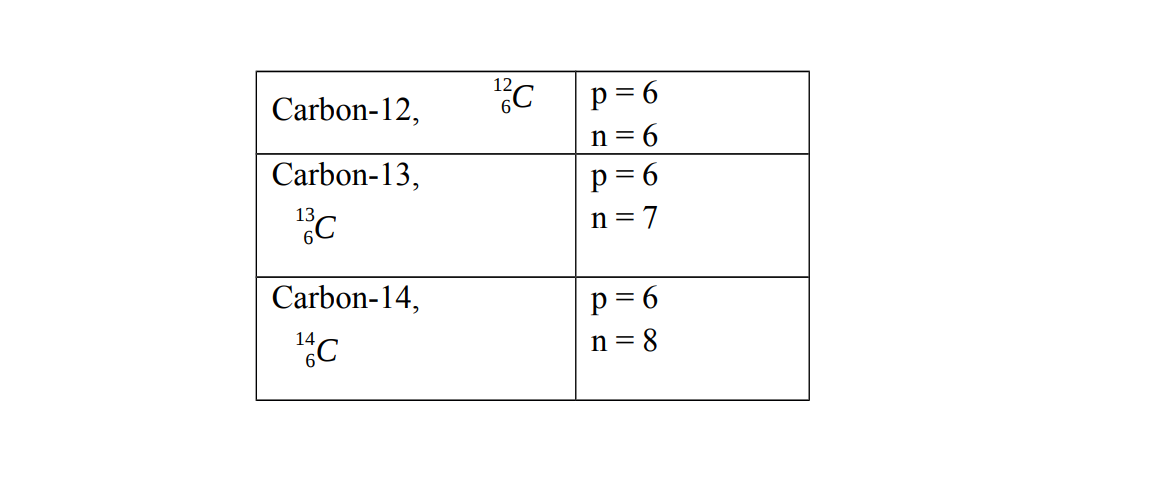##### Hydrogen has three isotopes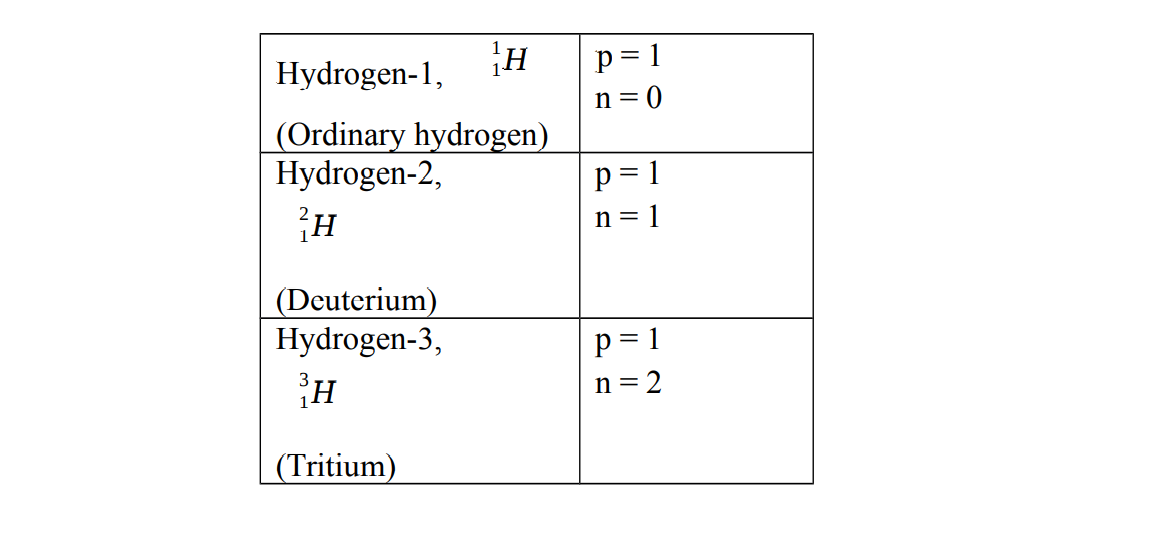##### Chlorine has two isotopes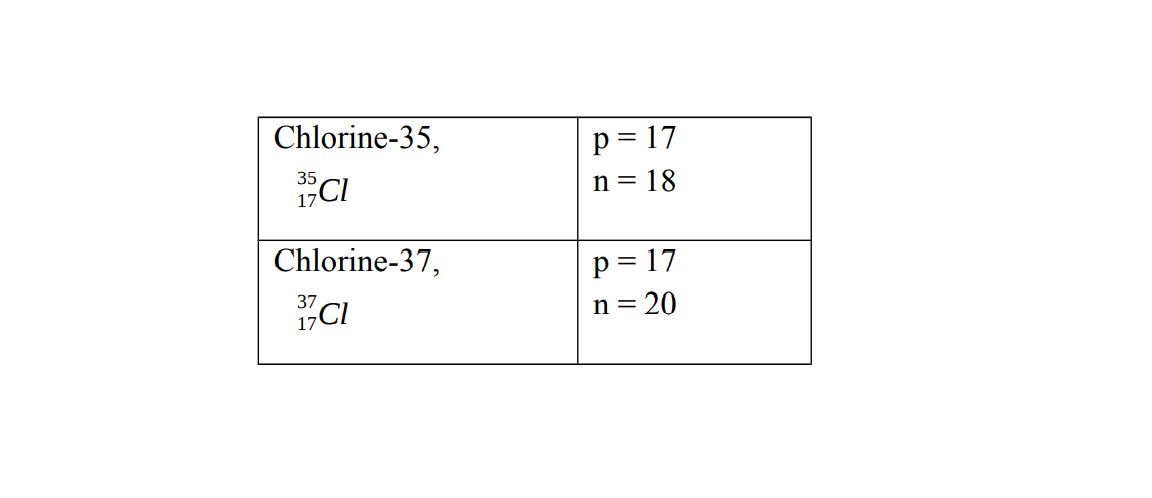#### use of Isotopes

• To check for leaks : Engineers can check oil and gas pipes for leaks by adding radioisotopes to the oil or gas. If a Geiger counter detects radiation outside the pipe, it means there is a leak. Radioisotopes used in this way are called tracers. Demonstrate the build-up of electrons in shells
• To treat cancer Radioisotopes can cause cancer. But they are also used in radiotherapy to cure cancer – because the gamma rays in radiation kill cancer cells more readily than healthy cells.
• To kill germs and bacteria Gamma rays kill germs too. So they are used to sterilize syringes and other disposable medical equipment. They also kill the bacteria that cause food to decay.

#### Mass spectrometer

The mass spectrometer is used for the separation of isotopes of an element and determination of their respective masses.

#### Relative atomic mass

Relative atomic mass of an element is the mass of one atom of an element compared to 1/12 the mass of carbon−12 isotope

Symbol: Ar

Formula: Relative atomic mass = $\frac{\mathrm{Mass number of isotope A x percentageabundance}}{100}$

#### Example 3

Chlorine has two main isotopes, ${}_{\mathrm{17}}{}^{\mathrm{35}}\mathrm{Cl}$ and ${}_{\mathrm{17}}{}^{\mathrm{37}}\mathrm{Cl}$ with abundances of 75% and 25% respectively. Calculate the relative atomic mass for chlorine.

Solution

Formula: $\frac{\mathrm{Mass Number of isotope A x percentageabundance}}{100}$

Relative atomic mass = $\frac{\mathrm{35 x percentageabundance}}{100}$ + $\frac{\mathrm{37 x percentageabundance}}{100}$

= $\frac{\mathrm{35 x 75}}{100}$ + $\frac{\mathrm{37 x 25}}{100}$

= $\frac{\mathrm{2625}}{100}$ + $\frac{\mathrm{925}}{100}$

= 26.25 + 9.25

Answer: Relative atomic mass = 35.5

Note The relative atomic mass of chlorine is 35.5 (not a whole number) because it is the average of two isotopes i.e. chlorine-35 and chlorine -37.

#### Electron shells

Alternative term: Energy levels. A shell is a concentric ring around the nucleus

The nucleus is also called the core of an atom.

The shells, in order of their increasing distance from the nucleus, are assigned by letters K, L, M, N etc.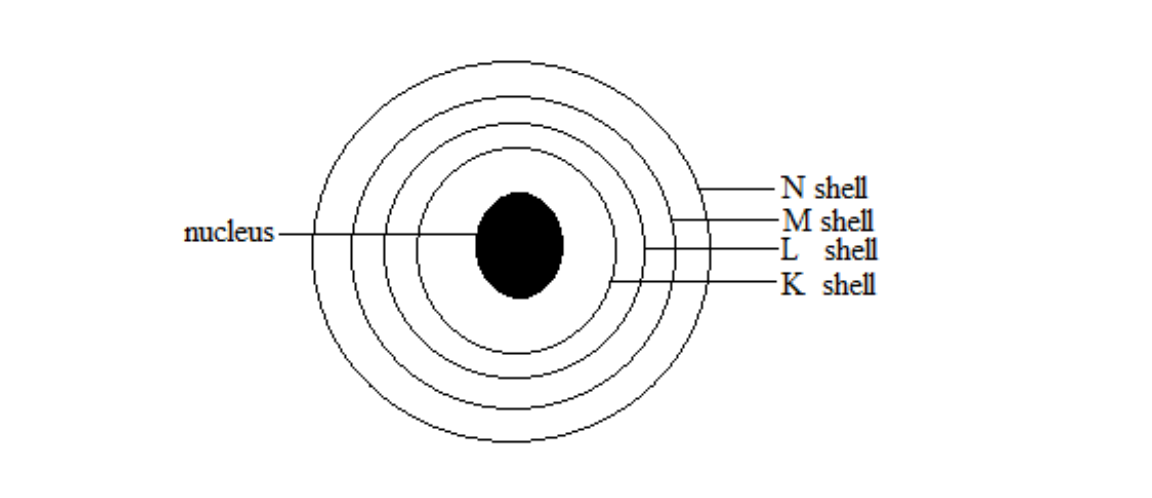#### Electron configuration

Alternative term: Electronic structure, Electron configuration is the arrangement of electrons in shells of atoms.

The maximum number of electrons each shell can accommodate is given by the formula: ${\mathrm{2n}}^{2}$ , where n is the number of shells.

##### The diagram below shows the number of electrons which an be accommodate for shell 1 and shell 2 namely K and L respectively

Note that n indicates the number of a shell in an electronic structure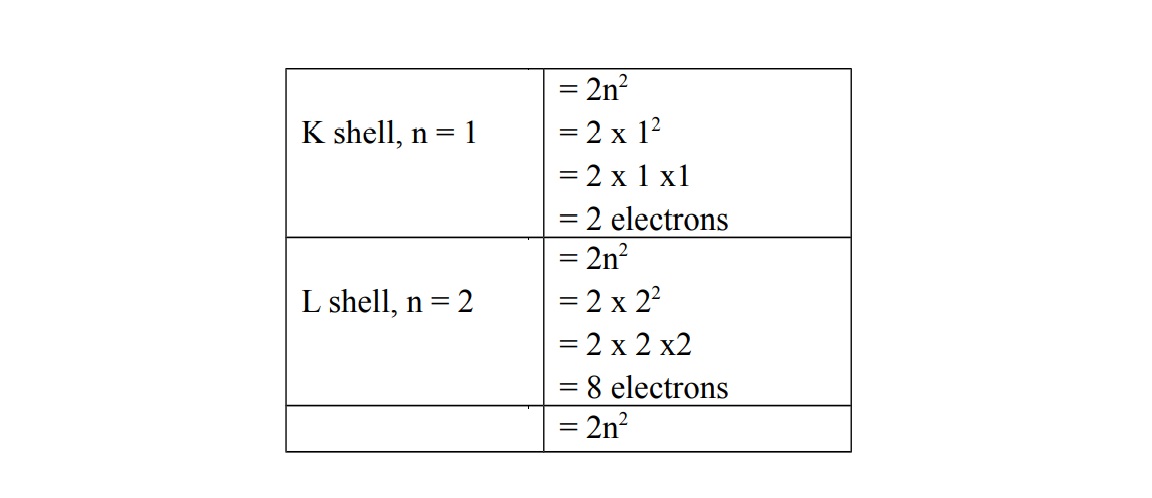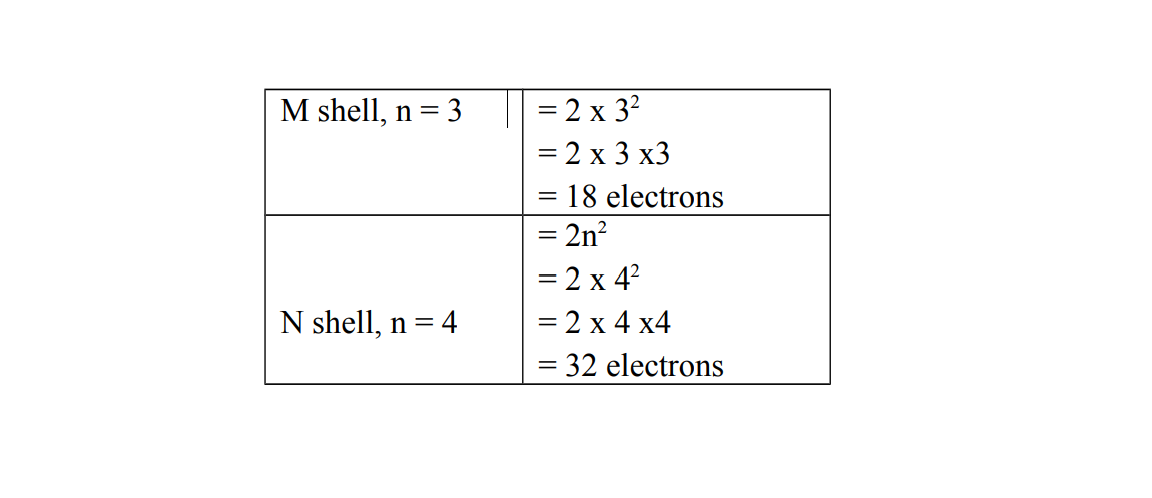Metals have 1 or 2 or 3 electrons in the outer most shells

Non-metals have 4 or 5 or 6 or 7 electrons in the outer most shell

The outer most shell is the last shell

#### Ways of showing the electron configuration by writing

The symbol of an element is written first followed by the number of electrons in each shell separated by a dot .

${}_{e}{}^{A}\mathrm{Cl}$

Cl = the name of the element, e = the number of electrons , A = the mass number

#### Example 4

Show by writing the electron configuration in each of the following nuclides:

a) ${}_{\mathrm{11}}{}^{\mathrm{23}}\mathrm{Na}$

b) ${}_{6}{}^{\mathrm{12}}C$

c) ${}_{\mathrm{17}}{}^{\mathrm{35.5}}\mathrm{Cl}$

Solution

(a) Na 2. 8. 1

(b) C 2.4

(c) Cl 2.8.7

#### Ways of showing the electron configuration by drawing

First show the electron configuration by writing, then draw the shells in form of rings or circles and indicate the number of electrons in each shell as crosses (x) or dots (∙)

#### Example 5

Show by drawing the electron configuration for each of the following nuclides:

a) ${}_{\mathrm{11}}{}^{\mathrm{23}}\mathrm{Na}$

b) ${}_{6}{}^{\mathrm{12}}C$

Solution